# Associated Legendre functions

(diff) ← Older revision | Latest revision (diff) | Newer revision → (diff)

The associated Legendre functions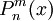$P^m_n(x)$ are polynomials which are most conveniently defined in terms of derivatives of the Legendre polynomials: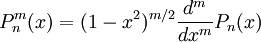$P^m_n(x)= (1-x^2)^{m/2} \frac{d^m}{dx^m} P_n(x)$

The first associated Legendre polynomials are: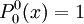$P_0^0 (x) =1$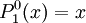$P_1^0 (x) =x$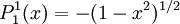$P_1^1 (x) =-(1-x^2)^{1/2}$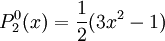$P_2^0 (x) =\frac{1}{2}(3x^2-1)$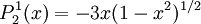$P_2^1 (x) =-3x(1-x^2)^{1/2}$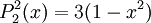$P_2^2 (x) =3(1-x^2)$

etc.